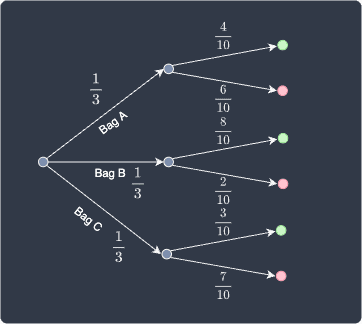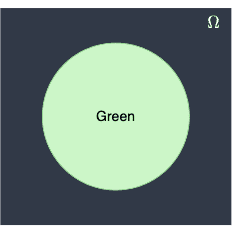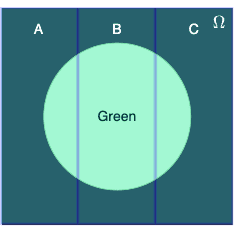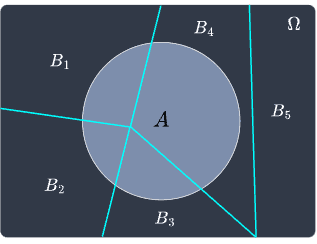search
Search
Map of Data Science
search toc
Thanks for the thanks!
close
Cancel
Post
account_circle
Profile
exit_to_app
Sign out
search
keyboard_voice
close
Searching Tips
Search for a recipe:
"Creating a table in MySQL"
Search for an API documentation: "@append"
Search for code: "!dataframe"
Apply a tag filter: "#python"
Useful Shortcuts
/ to open search panel
Esc to close search panel
to navigate between search results
d to clear all current filters
Enter to expand content previewDoc SearchCode Search BetaSORRY NOTHING FOUND!
mic
Start speaking...Voice search is only supported in Safari and Chrome.
Shrink
Navigate to
1. Basics of statistics
2. Basics of probability theory
3. Random variables
4. Point estimation
5. Discrete probability distributions
check_circle
Mark as learned
thumb_up
0
thumb_down
0
chat_bubble_outline
0
auto_stories new
settings

# Comprehensive Guide on Law of Total Probability

schedule Mar 5, 2023
Last updated
local_offer
Probability and Statistics
Tags
expand_more
map
Check out the interactive map of data science
Example.

# Motivating example

Suppose we have $3$ bags, with each bag containing $10$ balls:

• Bag $A$ has $6$ red balls and $4$ green balls.

• Bag $B$ has $2$ red balls and $8$ green balls.

• Bag $C$ has $7$ red balls and $3$ green balls.

A bag is randomly selected, and then a ball is randomly drawn. What is the probability that the drawn ball is green?

Solution. We can show the probability of every possible scenario using a probability tree diagram:We define the following events:

• events $A$, $B$ and $C$ denote choosing bags $A$, $B$ and $C$, respectively.

• events $\color{green}\text{green}$ and $\color{red}\text{red}$ denote choosing a green and red ball, respectively.

From the diagram, we can see that there are $3$ scenarios where we end up with a green ball:

• case 1 - we pick bag $A$, and then pick a green ball.

• case 2 - we pick bag $B$, and then pick a green ball.

• case 3 - we pick bag $C$, and then pick a green ball.

The probability that we draw a green ball is equal to the total sum of the probabilities that each of these cases would happen. The first case, for example, can be computed using the multiplication rulelink of probabilities:

$$\mathbb{P}(\text{case }1)= \mathbb{P}(A)\cdot \mathbb{P}({\color{green}\text{green}}|A)$$

Where $\mathbb{P}({\color{green}\text{green}}|A)$ is the probability that we select a green ball given that we selected bag $A$. Therefore, the probability of selecting a green ball is:

\begin{equation}\label{eq:xfN7kxAYwCrmDjDInlD} \begin{aligned}[b] \mathbb{P}({\color{green}\mathrm{green}}) &=\mathbb{P}(\text{case }1)+ \mathbb{P}(\text{case }2)+ \mathbb{P}(\text{case }3)\\ &=\mathbb{P}(A)\cdot\mathbb{P}({\color{green}\mathrm{green}}|A) +\mathbb{P}(B)\cdot\mathbb{P}({\color{green}\mathrm{green}}|B) +\mathbb{P}(C)\cdot\mathbb{P}({\color{green}\mathrm{green}}|C)\\ &=\frac{1}{3}\cdot\frac{4}{10}+\frac{1}{3}\cdot\frac{8}{10}+\frac{1}{3}\cdot\frac{3}{10}\\ &=0.5 \end{aligned} \end{equation}

This answers the question but let's now dig deeper into the underlying theory, particularly how this relates to the partitioning of the sample space.

## Partitioning of the sample space

The event that we draw a green ball can be illustrated as a Venn diagram:Because there are $3$ possible ways in which a green ball is drawn (via bag $A$, $B$ or $C$), we can consider the following partitions:The event $A\cap\color{green}\mathrm{green}$ represents the intersection between partition $A$ and the green circle. In this context, the intersection symbol $\cap$ is to be interpreted as "and". Notice how we obtain the entire green circle by taking the union of all the intersections of each partition and the green circle, that is:

$$\begin{equation}\label{eq:BHCuIgBSvU5xYYGmXEF} {\color{green}\mathrm{green}}= (A\cap{\color{green}\mathrm{green}})\cup (B\cap{\color{green}\mathrm{green}})\cup (C\cap{\color{green}\mathrm{green}}) \end{equation}$$

Note the following:

• $\mathbb{P}(A)+ \mathbb{P}(B)+ \mathbb{P}(C)=1$, that is, the probabilities of randomly selecting a bag add up to one. When this is true, we say that the partitions $A$, $B$ and $C$ constitute a sample space.

• the partitions do not necessarily have to be equally sized. In this case though, the partitions are of equal size since $\mathbb{P}(A) =\mathbb{P}(B) =\mathbb{P}(C)=1/3$.

Taking the probability of both sides of \eqref{eq:BHCuIgBSvU5xYYGmXEF} gives:

\begin{align*} \mathbb{P}({\color{green}\mathrm{green}}) &= \mathbb{P}\big[(A\cap{\color{green}\mathrm{green}})\cup (B\cap{\color{green}\mathrm{green}})\cup (C\cap{\color{green}\mathrm{green}})\big]\\ \end{align*}

Notice how three events $(A\cap{\color{green}\text{green}})$, $(B\cap{\color{green}\text{green}})$ and $(C\cap{\color{green}\text{green}})$ are mutually exclusive because they cannot happen simultaneously. Therefore, the third axiom of probabilitylink tells us that:

$$\begin{equation}\label{eq:dGnNFcZT5VuRfit3btu} \mathbb{P}({\color{green}\mathrm{green}}) = \mathbb{P}(A\cap{\color{green}\mathrm{green}})+ \mathbb{P}(B\cap{\color{green}\mathrm{green}})+ \mathbb{P}(C\cap{\color{green}\mathrm{green}}) \end{equation}$$

Now, recall the multiplication rule of probabilities, which is simply a rearranged version of the formula of conditional probability:

$$\mathbb{P}(E_1\cap{E_2}) =\mathbb{P}(E_1)\cdot\mathbb{P}(E_2\vert{E_1})$$

Where:

• $E_1$ and $E_2$ are some events.

• $\mathbb{P}(E_2\vert{E_1})$ is the probability of $E_2$ occurring given $E_1$ has occurred.

Applying this rule to \eqref{eq:dGnNFcZT5VuRfit3btu} gives:

\begin{equation}\label{eq:X25GeWfNWHCVDYIhCQm} \begin{aligned}[b] \mathbb{P}({\color{green}\mathrm{green}})&=\mathbb{P}(A\cap{\color{green}\mathrm{green}}) +\mathbb{P}(B\cap{\color{green}\mathrm{green}}) +\mathbb{P}(C\cap{\color{green}\mathrm{green}})\\ &=\mathbb{P}(A)\cdot\mathbb{P}({\color{green}\mathrm{green}}|A) +\mathbb{P}(B)\cdot\mathbb{P}({\color{green}\mathrm{green}}|B) +\mathbb{P}(C)\cdot\mathbb{P}({\color{green}\mathrm{green}}|C) \end{aligned} \end{equation}

This is exactly the same formula \eqref{eq:xfN7kxAYwCrmDjDInlD} we used earlier! This formula is called the law of total probability.

Theorem.

# Law of total probability

If the events $B_1$, $B_2$, $\cdots$, $B_k$ constitute a partition of the sample space $S$ such that $\mathbb{P}(B_i)\ne0$ for $i=1$, $2$, $\cdots$, $k$, then for any event $A$ of $S$, we have that:

$$\mathbb{P}(A) =\sum^k_{i=1}\mathbb{P}(A\cap{B_i}) =\sum^k_{i=1}\mathbb{P}(B_i)\cdot\mathbb{P}(A|B_i)$$

The law of total probability is sometimes known as the rule of elimination.

Proof. The flow of the proof is very similar to how we solved the example problem earlier. Suppose events $B_1$, $B_2$, $\cdots$, $B_k$ constitute a partition of the sample space $S$, that is:

$$\mathbb{P}(B_1) + \mathbb{P}(B_2) + \cdots + \mathbb{P}(B_k) = 1$$

For instance, for $k=5$, the Venn diagram may look like the following:Notice how not all partitions need to intersect with event $A$. Now, event $A$ can be constructed by summing up all the intersection between $A$ and the partitions $B_1$, $B_2$, $\cdots$, $B_k$, that is:

\begin{align*} A&=(B_1\cap{A})\cup(B_2\cap{A})\cup\cdots\cup(B_k\cap{A}) \end{align*}

Taking the probability of both sides yields:

\begin{align*} \mathbb{P}(A)&=\mathbb{P}\big[(B_1\cap{A}) \cup(B_2\cap{A})\cup\cdots\cup(B_k\cap{A})\big] \end{align*}

Because the events $(B_i\cap{A})$ where $i=1$, $2$, $\cdots$, $k$ are disjoint, the third axiom of probabilitylink tells us that:

$$\mathbb{P}(A) =\mathbb{P}(B_1 \cap {A}) + \mathbb{P}(B_2 \cap {A}) + \cdots + \mathbb{P}(B_k \cap {A})$$

Using the multiplication rule, that is $\mathbb{P}(B\cap{A}) =\mathbb{P}(B) \cdot\mathbb{P}(A|B)$, we have the following:

\begin{align*} \mathbb{P}(A)&= \mathbb{P}(B_1)\cdot\mathbb{P}(A|B_1) +\mathbb{P}(B_2)\cdot\mathbb{P}(A|B_2) +\cdots+\mathbb{P}(B_k)\cdot\mathbb{P}(A|B_k)\\ &=\sum^k_{i=1}\mathbb{P}(B_i)\cdot\mathbb{P}(A|B_i) \\ \end{align*}

This completes the proof.

As a reference for the latter guides, we will also state the law of total probability when there are only two events $B_1$ and $B_2$ that partition the sample space.

Theorem.

# Law of total probability for two events

If events $B_1$ and $B_2$ constitute a partition of the sample space $S$ such that $\mathbb{P}(B_1)\ne0$ and $\mathbb{P}(B_2)\ne0$, then for any event $A$ of $S$, we have that:

$$\mathbb{P}(A) = \mathbb{P}(A|B_1)\cdot\mathbb{P}(B_1) + \mathbb{P}(A|B_2)\cdot\mathbb{P}(B_2)$$
thumb_up
thumb_down
Comment
Citation
Ask a question or leave a feedback...
thumb_up
0
thumb_down
0
chat_bubble_outline
0
settings
Enjoy our search
Hit / to insta-search docs and recipes!